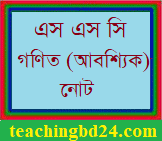SSC Mathematics Note 14th Chapter Ratio, Similarity, and Symmetry

SSC Mathematics Note 14th Chapter Ratio, Similarity, and Symmetry. For comparing two quantities,   their ratios are to be considered.  Again,  for determining ratios, the two quantities are to be measured in the same units.  In algebra, we have discussed this in detail. A polygon is a closed figure made of several line segments. A polygon is said to be regular if all its sides are of equal length and all its angles are equal. The triangle is a polygon made up of the least number of line segments.

SSC Mathematics Note 14th Chapter Ratio, Similarity, and SymmetryAn equilateral triangle is a regular polygon of three sides. An equilateral triangle is regular because its sides, as well as angles, are equal. A square is the regular polygon of four sides. The sides of a square are equal and each of the angles is equal to one right angle. Similarly, in regular pentagons and hexagons, the sides are equal and the angles are equal as well.

teachingbd24.com is such a website where you will get all kinds of necessary information regarding educational notes, suggestions and question patterns of schools, colleges, and madrasas. Particularly, you will get here special notes of physics that will be immensely useful to both students and teachers. The builder of the website is Mr. Md. Shah Jamal who has been serving for 32 years as an Assistant Professor of Physics at BAF Shaheen College Dhaka. He expects that this website will meet up all the needs of Bengali version learners /students. He has requested concerned students and teachers to spread this website home and abroad.